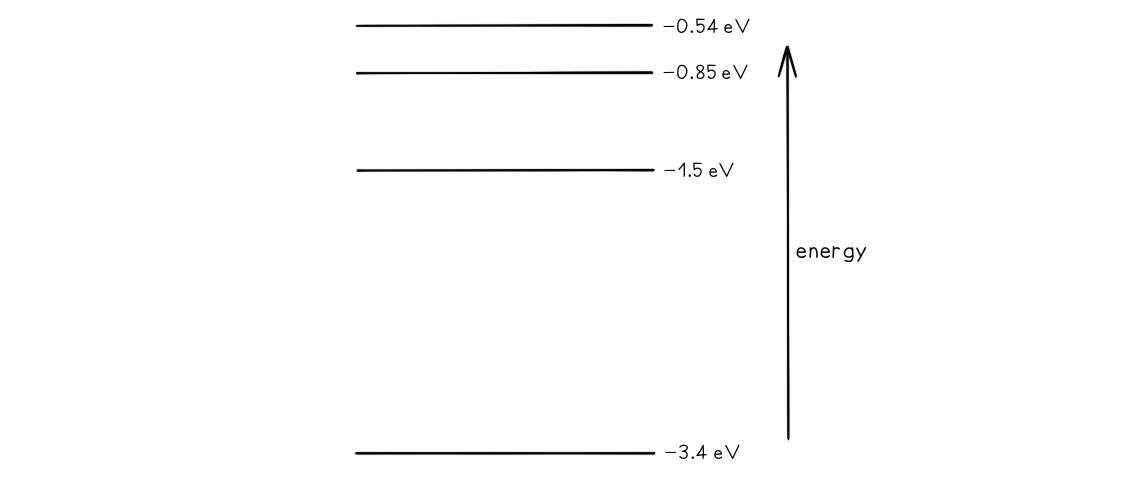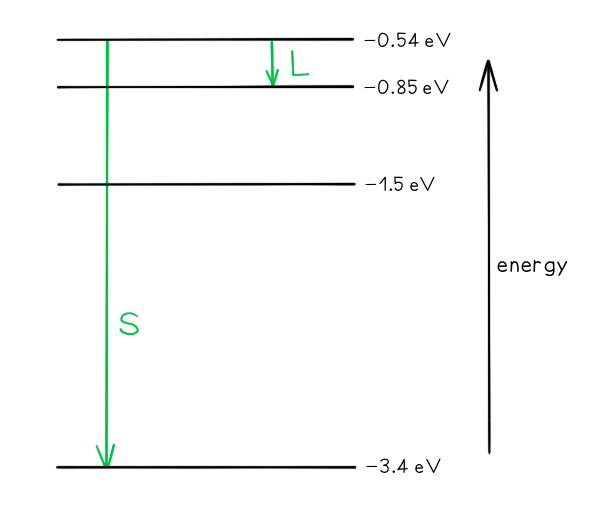# CIE A Level Physics复习笔记22.3.3 Calculating Discrete Energies

### Calculating Discrete Energies

• The difference between two energy levels is equal to a specific photon energy
• The energy (hf) of the photon is given by:

ΔE = hf = E2 - E1

• Where,
• E1 = Energy of the higher level (J)
• E2 = Energy of the lower level (J)
• h = Planck’s constant (J s)
• f = Frequency of photon (Hz)
• Using the wave equation, the wavelength of the emitted, or absorbed, radiation can be related to the energy difference by the equation:• This equation shows that the larger the difference in energy of two levels ΔE , the shorter the wavelength λ and vice versa

#### Worked Example

Some electron energy levels in atomic hydrogen are shown below.The longest wavelength produced as a result of electron transitions between two of the energy levels is 4.0 × 10–6 m.a) Draw and mark:

• The transition giving rise to the wavelength of 4.0 × 10–6 m with letter L.
• The transition giving rise to the shortest wavelength with letter S.

b) Calculate the wavelength for the transition giving rise to the shortest wavelength.

Part (a)Photon energy and wavelength are inversely proportional, so the largest energy change corresponds to the shortest wavelength (line S) and the smallest energy change corresponds to the longest wavelength (line L)

Part (b)

Step 1:            Write down the equation linking the wavelength and the energy levelsStep 2:            Identify the energy levels giving rise to the shortest wavelength

E1 = 0.54 eV

E2 = 3.4 eV

Step 3:            Calculate the wavelength

To convert from eV → J: multiply by 1.6 × 10-19